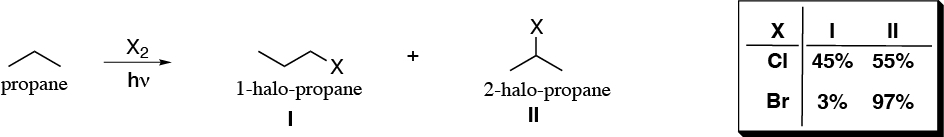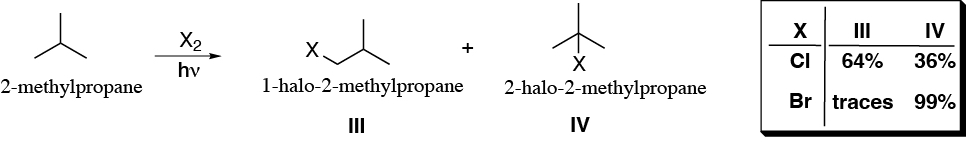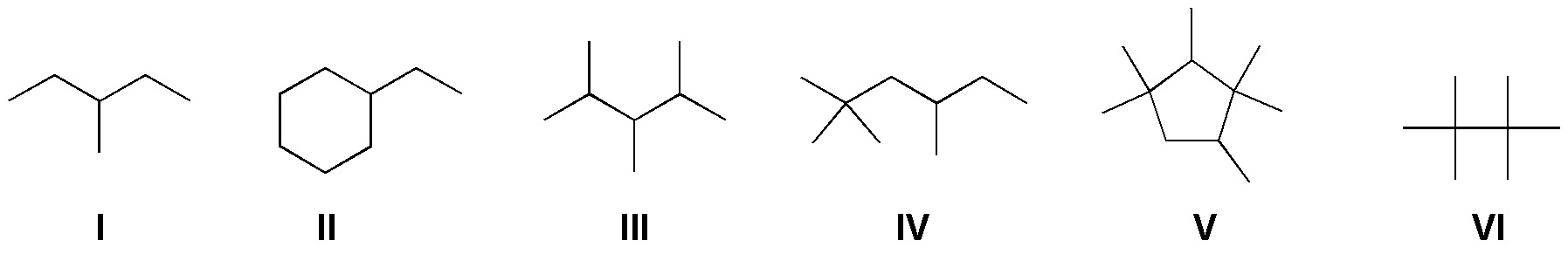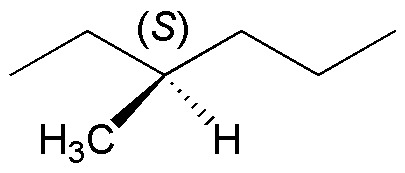# Alkanes – Organic Chemistry – list of solved problems

Go to the page with the solutions to the problems.

## Alkanes – list of problems

### Problem 1:

(a) Draw and name all possible isomers of molecular formula C5H12.

(b) Identify the primary, secondary and tertiary hydrogens in each of the structures.

(c) Deduce which isomer forms a single compound in the monobromination reaction, without considering possible stereoisomers.

### Problem 2:

Draw the profile of the chlorination reaction via radical of methane, including the most likely transition state that would be observed in such a profile.

### Problem 3:

The halogenation reaction via radical of propane and 2-methylpropane gives the products and percentages indicated. Justify these experimental results and determine the relative reactivities of each type of hydrogen as a function of the halogen used.### Problem 4:

In view of the results of problem 3, predict what proportion of products will be obtained in the monochlorination of 2,3-dimethylbutane.

### Problem 5:

Assuming that the order of reactivity of the hydrogens in the bromination reaction is:

• 3º 1600
• 2º 82
• 1º 1

predict the major product in the monobromination reaction of propane, butane, 2-methylpropane, cyclopentane and methylcyclopentane.

### Problem 6:

State the major product(s) of the monobromination of the following compounds:### Problem 7:

Draw the constitutional isomers resulting from the monochlorination of 2,4-dimethylpentane, indicating which ones present chiral carbons.

### Problem 8:

Assuming that the chlorination reaction of methylpropane with an excess of chlorine leads to the formation of a dichlorinated derivative, draw all the possible constitutional isomers that would be obtained.

### Problem 9:

The bromination of pentane leads to the formation of three products one of which is a racemic form. Describe each of them and justify the result.

### Problem 10:

Assuming in the racemic mixture of the previous problem a second bromination occurs, draw the most probable structure of the products obtained, justifying the result.

### Problem 11:

Starting from (S)3-methylhexane, when the radical monobromination reaction is carried out, a reaction crude is obtained which is optically inactive. Justify the result obtained.### Problem 12:

If cyclobutane reacts with more than one mole of bromine, how many dibromocyclobutanes would be obtained?

### Problem 13:

Draw the structures of the hydrocarbons (alkanes) that would be obtained in the pyrolysis of heptane.

### Problem 14:

What are the products of complete combustion of n-pentane? Adjust the reaction.

### Problem 15:

Adjust the complete combustion reaction of n-hexane.

### Problem 16:

Adjust the partial combustion reaction of isooctane (CH3 )3CC(CH3 )3.

### Problem 17:

Draw all the constitutional isomers (disregarding stereoisomers), obtained in the monochlorination of the following compounds:

(a) butane

(b) 2-methylbutane

(c) 2,3-dimethylbutane

(d) 3-methylpentane

Indicate in what proportion each of them would be formed taking into account the relative reactivities indicated in problem 3.

### Problem 18:

Draw all the constitutional isomers, disregarding stereoisomers, obtained in the monochlorination of the following compounds:

(a) 2,2-dimethylbutane

(b) 2,2-dimethylpropane

(c) n-hexane

(d) methylcyclohexane

(e) 1,4-dimethylcyclohexane

### Problem 19:

Identify the products obtained in the monochlorination of 2,4-dimethylpentane. Indicate whether any of these compounds have chiral carbons. If the rotational power of the reaction crude is determined, what would be the result?

### Problem 20:

Identify the products obtained in the dibromination of 2,4-dimethylpentane. Indicate if any of these compounds have chiral carbons.

### Problem 21:

Calculate the relative reactivity of the hydrogen atoms of butane in the chlorination reaction, knowing that monochlorination of this hydrocarbon yields 1-chlorobutane (28%) and 2-chlorobutane (72%).

### Problem 22:

Calculate the relative reactivity of the hydrogen atoms of 2-methylbutane in the chlorination reaction, knowing that monochlorination of this hydrocarbon leads to the formation of 1-chloro-2-methylbutane (27%), 2-chloro-2-methylbutane (23%), 2-chloro-3-methylbutane (36%) and 1-chloro-3-methylbutane (14%).

### Problem 23:

From the following relative reactivity data [ 1 : 1 : 4 : 6 ] of the following types of hydrogens [(CH3-)3 : (CH3-)2 : CH2– : CH- ], indicate the products obtained and the expected percentage in the monochlorination of 2,2,4-trimethylpentane.

### Problem 24:

Predict what will be the major product(s) of the monobromination reaction for the following alkanes:

(a) methylcyclopentane

(b) 2-methylpentane

(c) 2,2,5-trimethylhexane

(d) methylcyclopropane

(e) isopentylcyclopentane.

### Problem 25:

Assuming you have to prepare a monobrominated derivative by a radical reaction, which hydrocarbons would you choose from the following list, and which would you discard?

(a) methylcyclohexane

(b) 2-methylpropane

(c) 2,2-dimethylpropane

(d) 2,3-dimethylbutane

(e) 2,4-dimethylpentane

(f) cycloheptane.

### Problem 26:

The bromination reaction of both 2-methylbutane and (R)-3-methylhexane leads in both cases to a reaction crude that is optically inactive. Justify the result.

### Problem 27:

Starting from propane, pentane and 2,3-dimethylbutane, draw all the possible alkanes that can be formed by combination of the radicals obtained in a pyrolysis reaction.

### Problem 28:

Adjust the complete combustion reaction of n-butane.

### Problem 29:

Fit the partial combustion reaction (CO + H2O) of n-dodecane.

Shares